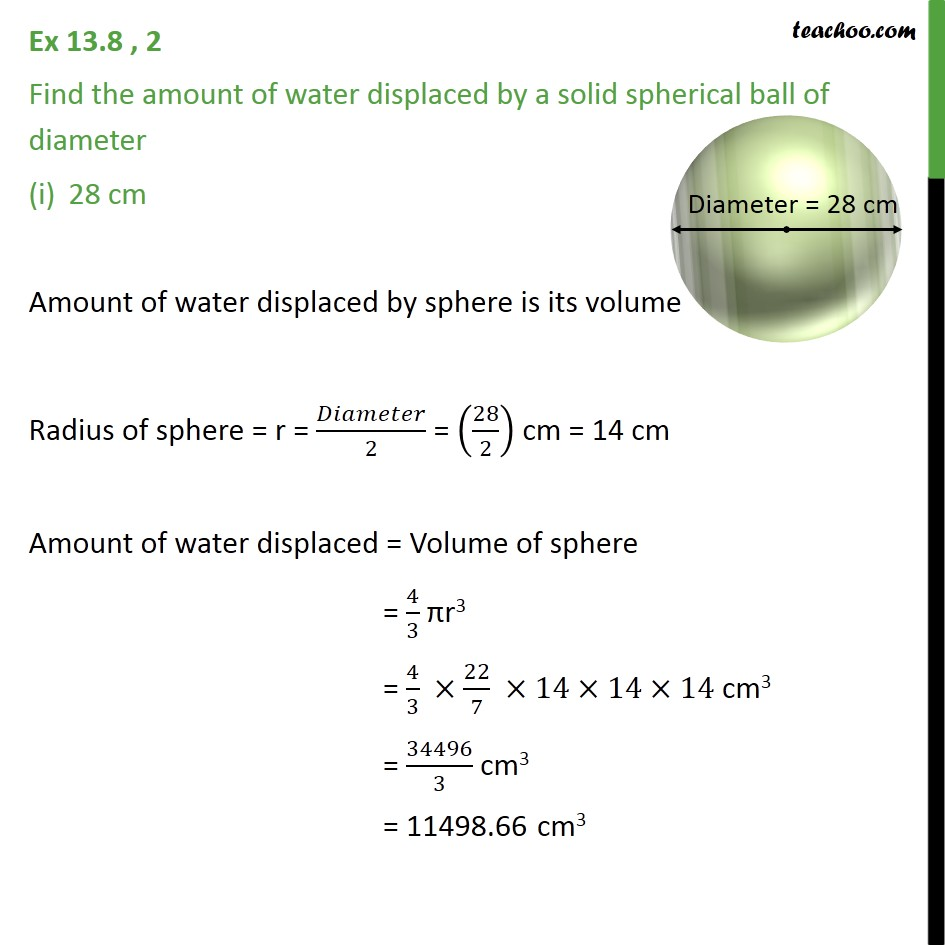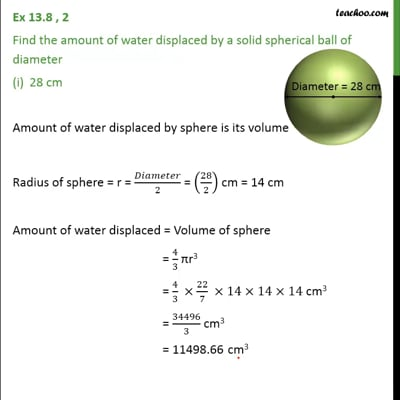Ex 13.8

Chapter 13 Class 9 Surface Areas and Volumes (Term 2)
Serial order wiseThis video is only available for Teachoo black users

### Transcript

Ex 13.8 , 2 Find the amount of water displaced by a solid spherical ball of diameter 28 cm Amount of water displaced by sphere is its volume Radius of sphere = r = /2 = (28/2) cm = 14 cm Amount of water displaced = Volume of sphere = 4/3 r3 = 4/3 22/7 14 14 14 cm3 = 34496/3 cm3 = 11498.66 cm3